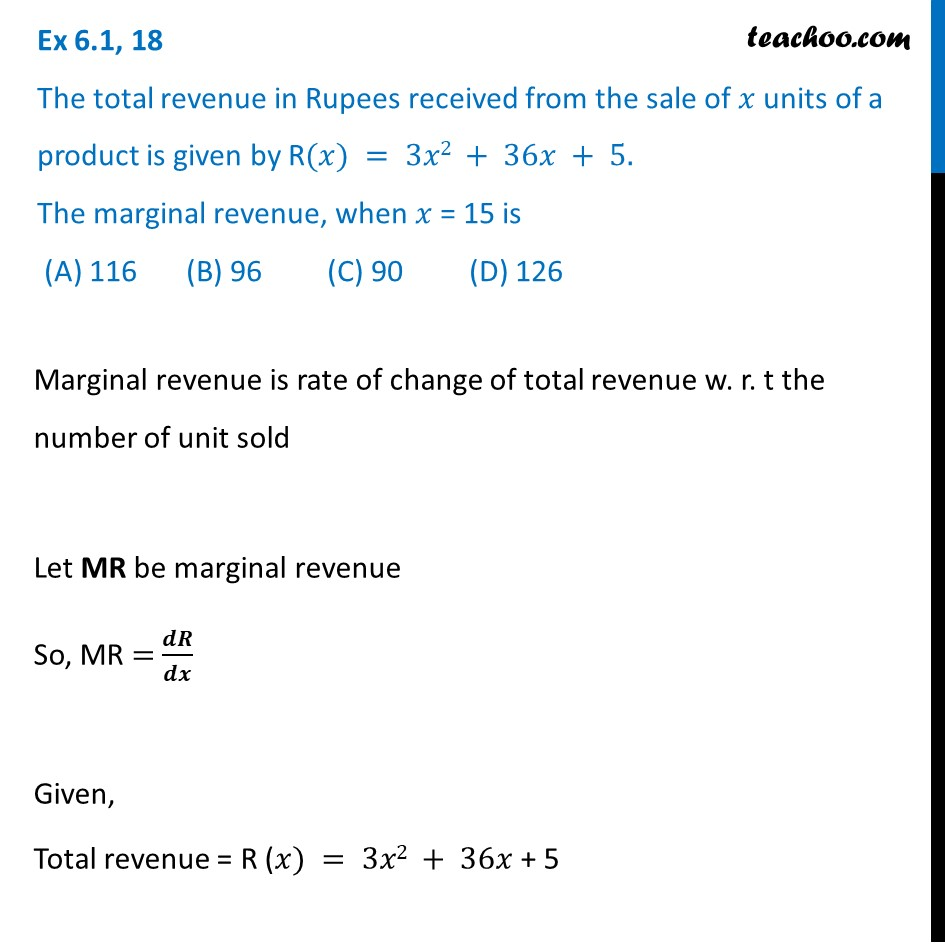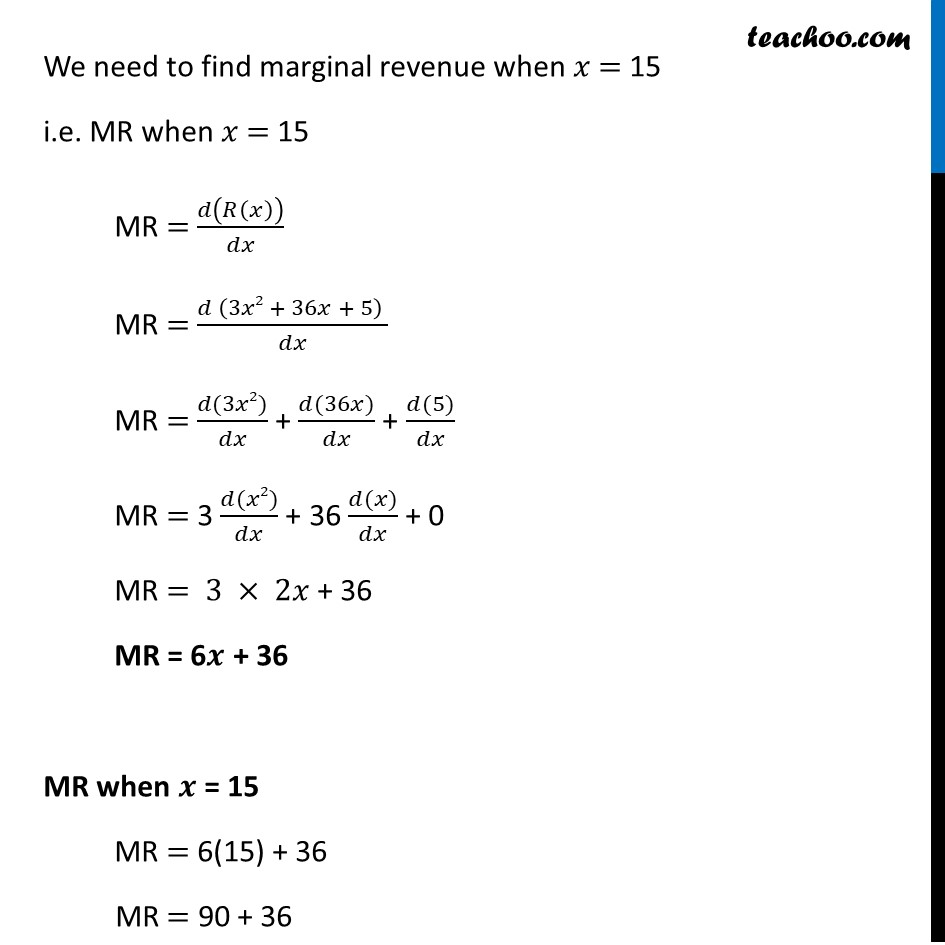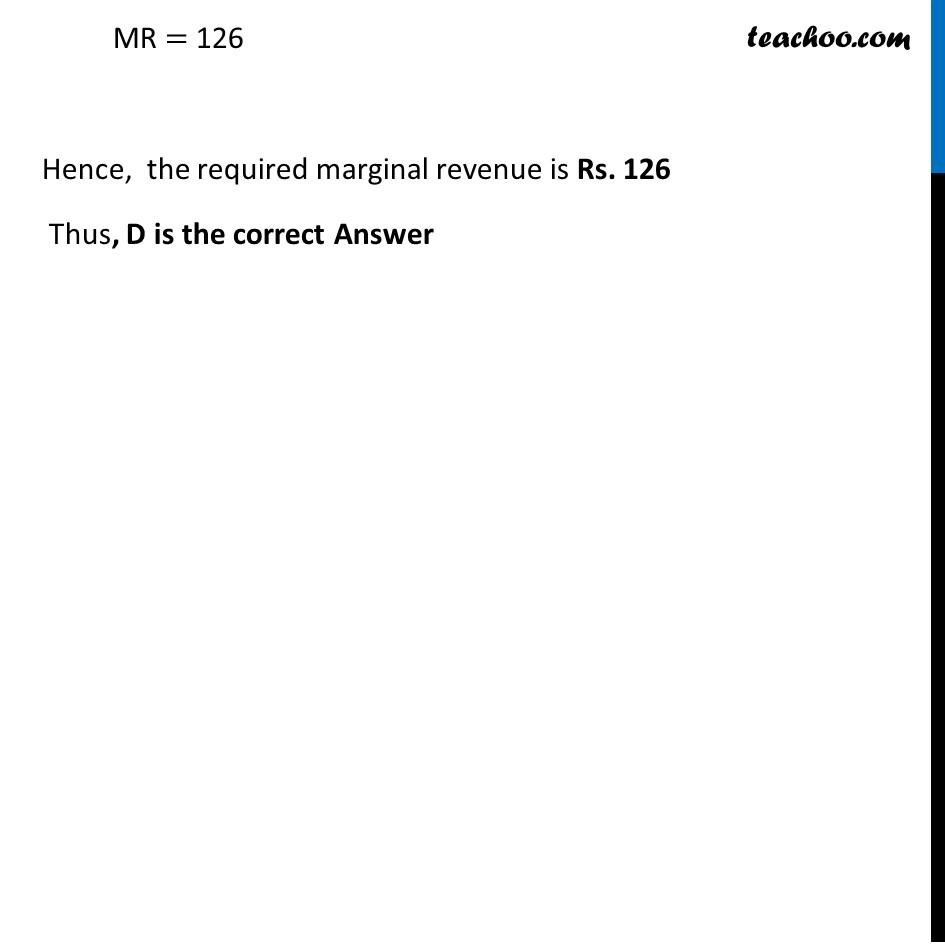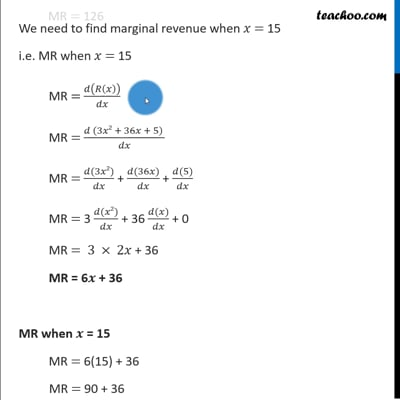Finding rate of change

Chapter 6 Class 12 Application of Derivatives
Concept wiseThis video is only available for Teachoo black users

Learn in your speed, with individual attention - Teachoo Maths 1-on-1 Class

### Transcript

Ex 6.1, 18 The total revenue in Rupees received from the sale of 𝑥 units of a product is given by R(𝑥) = 3𝑥2 + 36𝑥 + 5. The marginal revenue, when 𝑥 = 15 is (A) 116 (B) 96 (C) 90 (D) 126Marginal revenue is rate of change of total revenue w. r. t the number of unit sold Let MR be marginal revenue So, MR = 𝒅𝑹/𝒅𝒙 Given, Total revenue = R (𝑥) = 3𝑥2 + 36𝑥 + 5 We need to find marginal revenue when 𝑥 = 15 i.e. MR when 𝑥 = 15 MR = 𝑑(𝑅(𝑥))/𝑑𝑥 MR = (𝑑 (3𝑥2 + 36𝑥 + 5) )/𝑑𝑥 MR = (𝑑(3𝑥2))/𝑑𝑥 + (𝑑(36𝑥))/𝑑𝑥 + (𝑑(5))/𝑑𝑥 MR = 3 (𝑑(𝑥2))/𝑑𝑥 + 36 (𝑑(𝑥))/𝑑𝑥 + 0 MR = 3 × 2𝑥 + 36 MR = 6𝒙 + 36 MR when 𝒙 = 15 MR = 6(15) + 36 MR = 90 + 36 MR = 126 Hence, the required marginal revenue is Rs. 126 Thus, D is the correct Answer